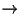Next: 15.1.2 Evaluation measuers Up: 15.1 Some Background on Previous: 15.1 Some Background on

## 15.1.1 Common design features of IR systems

• invsersed index
$BE>CO(B index, $BK\$N:w0z(B • position information $B@vN}$5$l$?(B inverted index $B$OC18l$NM-L5$NB>$K(B $B0LCV>pJs$bJ];}$9$k(Bphrases $B8!:w$,2DG=(B
• car insurance'' $B$H$$&(B query BKBP7F(B, B8l,3N(B B=gGJBsG=P8=9k(B document B[&,(B,Bhj(B relevant • BC1Jk0LCV@1@H(B car insurance rates'' BH(B rates for car insurance'' Br(B BF10lNbNH07(J$$(BNLP reserch $B$NJ,Ln(B
• $B8=:_$N(B IR system $B$NB?$/$O(B phrases $B$r(B pharses $B$H$7$F(B $B07$&$N$G$O$J$/(B, word $BC10L$G07$&(B(n-gram $BEy(B)$B$=$l$J$j$N(B performance • collocations $B$NLdBj(B • stop list • from $B$d(B could $B$J$I$N(B function words($B5!G=8l(B)$B$O(B, word-by-word $B$J8!:w$K$OITMW(B.
stop list $B$H$7$FJ];}$7$F$*$-(B, inveted index $B$KEPO?$7$J$$(B. (Table 15.1 BK(B stop list BNNc(B) • inverted index BN(B sizeBrBgI}K8:i93H,G-k(B (Zipf BNK!B'(B..) • phrases B8!:wKO(B function words BOI,MW(BBB?/N8!:w(B engines BO(Bstop list Br;HoJ$$(B
• stemming
• laughing,laugh,laughs,laughed $B$rF10l;k$7$?$$(B • suffix BNJQ2=r5[<}9k(B • Lovins and Porter stemmers B,0lHLE*(B • B3liN(B stemmer BO(B B8lNI=AX7+8+J$$$N$G0UL#E*$J6hJL$r(B $B9T$&$3$H$,$G$-$J(B
(gallery $B$H(B gall $B$O(B $B$*$J$8(B gall- $B$K(B stemming $B$5$l$k(B)

1999-08-03